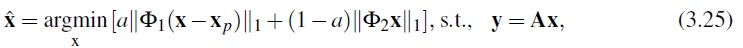CT image recovery from incomplete projections is an ill-conditioned problem without a unique solution. Classically, to be able to recover images from incomplete data, different prior models of the images have been used in statistical iterative reconstruction algorithms. However, after the introduction of CS, the research focus changed. Currently, the focus is to recover high quality low dose images from fewer projection angles, as demonstrated in figure 3.4. Two different approaches are currently used for employing CS in CT imaging, depending on
the primary objective, and these are as follows:
1) Radiation dose reduction: to reduce the X-ray dose, CS-based CT reconstruction algorithms are used to reconstruct high quality CT images either from few high dose projections or from many low dose projections. However, to date, there is no clear evidence as to which approach is best.
2) Motion artifact reduction: CS improves the temporal resolution of the CT imaging by reducing the number of projections or by limiting the projection angle. This higher temporal resolution directly improves the performance of CT scanners for imaging moving objects, i.e., respiratory motions in chest CT scans and cardiac motions in cardiac-CT.

Current CS-based reconstruction algorithms suffer from two drawbacks: their high computational load makes them impracticable for routine clinical use  and the CT statistics and scanning geometries have not been incorporated in the problem formulation. As a result, there are considerable hurdles to be overcome for these methods to be used in clinical CT systems. The main challenge in solving CS optimization problem within a reasonable amount of time in CT imaging arises from the size of the measurement matrix A.
Currently, in most available CS-based reconstruction methods used for modern CT geometries the measurement matrix A is a Radon sampling matrix, similar to ART, which models the rays going through the patient. To reconstruct a 512*512 pixel image from 900 sensors and 1200 projection angles, A would be a 1080000*262144 matrix. As typical iterations each usually require two multiplications by A and AT , it would take several hours of computation on a typical desktop computer to reconstruct the image.

To use CS in CT imaging, the measurements need to be maximally sensitive to as many of the used dictionary basis as possible. Moreover, the images that differ in a small number of basis must be as distinguishable as possible in measurement space. Therefore, optimal measurements are as unstructured as possible in terms of their sensitivity to basis images, but they should also maximally separate sparse representations in measurement space. This property is quantitatively measured by incoherency of the measurement basis. However, the measurement techniques in CT imaging are not very flexible, due to the rotational geometry. These limitations increase the coherency between the measurements, which increases the number of samples needed by CS in CT reconstruction . To reduce the number of needed samples two approaches have been used:  1) utilization of better sparsifying methods, such as group sparsity , and 2) improving the measurement matrices to reduce the coherency of the measurements .
As an example of the first group, Prior Image Constrained Compressed Sensing (PICCS) uses a prior image xp in its formulation to represent the image with more sparsity. The prior image xp is a low quality image computed by smoothing the image reconstructed using FBP from few projections. To reconstruct the CT images, PICCS uses the following optimization expression,in which F1 and F2 are two sparsifying transforms, and a is a positive constant. In PICCS, total variation is used for both F1 and F2 and, in addition, a = 0:91. Equation (3.25) is solved based on an ART reconstruction followed by a TV denoising. In each iteration ART is used to recover the image from y = Ax, then a steepest descent algorithm is used to minimize the total variation of the weighted summation in equation (3.25).
To reduce the coherency of the measurements in  an improved detector arrangement, as shown in figure 3.5, was proposed, but not tested. In  different sampling strategies, as shown in figure 3.6, are investigated for tomography. It has been shown that random view angle sub-sampling and uniform view sub-sampling are not very different. However, random detector sub-sampling has improved the incoherency. Using this property, coded aperture Xray imaging , as shown in figure 7, has been proposed that optimizes the CT hardware for CS application.Figure 5: Angular independent coded aperture CT. The white regions indicate the sampled
part of the data. Reproduced, with permission, fromFigure 3.6: Different sampling strategies being considered, as demonstrated on a fan-beam geometry. The blue circles denote source locations (corresponding to view angles), and lines denote source-detector pairs (for clarity, only the lines for one source location are shown for each case). The solid and dashed lines correspond to measured and non-measured line integrals, respectively. The large circle represents the source trajectory, and the small circle represents the imaged domain. (A) Uniform view sub-sampling, (B) random view angle subsampling, (C) uniform detector sampling, and (D) random detector sub-sampling. Reproduced, by permission, from .Figure 3.7: Schematic of a coded aperture CT scanner proposed in . Black regions block the X-ray rays. Reproduced, with permission, from .